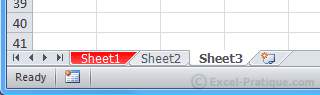# VBA Course: Colors

Let's start by assigning a color to the text in A1.

After adding Font., we get this result: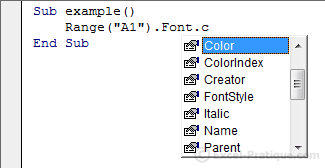There are two different ways that we can set the color: ColorIndex, which has 56 colors, or Color which makes it possible to use any color at all.

## ColorIndex

Here you can see the 56 colors that are available through ColorIndex: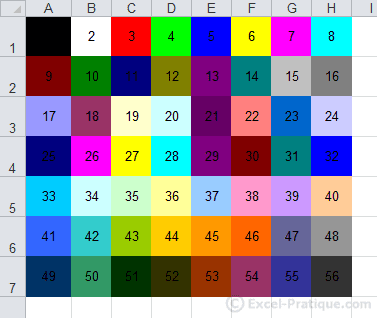To set the color of our text to one of these 56, we should write:

``````Sub example()
'Text color for A1: green (Color num. 10)
Range("A1").Font.ColorIndex = 10
End Sub``````

This code will give us the following result: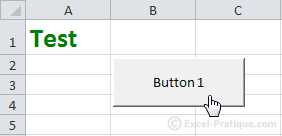For versions of Excel lower than 2007: using ColorIndex is preferable to using Color.

## Color

Here is a similar example in which we use Color:

``````Sub example()
'Text color for A1: RGB(50, 200, 100)
Range("A1").Font.Color = RGB(50, 200, 100)
End Sub``````

In this case, the color is: RGB(50, 200, 100).

RGB stands for Red-Green-Blue, and the numerical values go from 0 to 255 for each color.

A few examples of colors so that you can understand this better:

• RGB(0, 0, 0): black
• RGB(255, 255, 255): white
• RGB(255, 0, 0): red
• RGB(0, 255, 0): green
• RGB(0, 0, 255): blue

Luckily, there are lots of easy ways to find the RGB values for colors. Here's one: RGB Values

Choose the color that you want and just copy the three values into the RGB(red_value, green_value, blue_value).

So to change our text color in purple, we should use the following code:

``````Sub example()
'Text color for A1: RGB(192, 32, 255)
Range("A1").Font.Color = RGB(192, 32, 255)
End Sub``````

This code will produce the following result: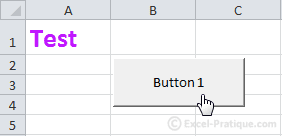For versions of Excel lower than 2007: the number of colors is limited (the closest available color to the RGB values will be used).

We will now create a macro that adds a border to the active cell using ActiveCell.

The border will be heavy and red:

``````Sub example()
'Border weight
ActiveCell.Borders.Weight = 4
'Border color: red
ActiveCell.Borders.Color = RGB(255, 0, 0)
End Sub``````

Result: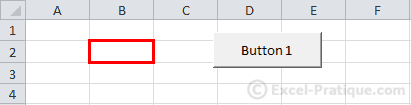To apply this effect to many cells at once, we can use the Selection command:

``````Sub example()
'Border weight
Selection.Borders.Weight = 4
'Border color: red
Selection.Borders.Color = RGB(255, 0, 0)
End Sub``````

## Add background color to the selected cells

``````Sub example()
'Add background color to the selected cells
Selection.Interior.Color = RGB(174, 240, 194)
End Sub``````

Result: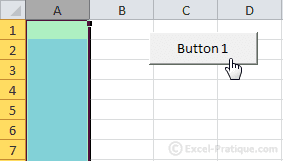## Add color to the tab for a worksheet

``````Sub example()
'Add color to the tab for "Sheet1"
Sheets("Sheet1").Tab.Color = RGB(255, 0, 0)
End Sub``````

Result: